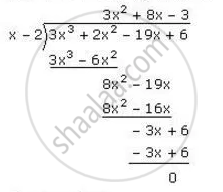Share

Using the Remainder Theorem, Factorise Each of the Following Completely. 3x3 + 2x2 − 19x + 6 - ICSE Class 10 - Mathematics

Question

Using the Remainder Theorem, factorise each of the following completely.

3x+ 2x2 − 19x + 6

Solution

For x=2, the value of the given

expression 3x^3+2x^2-19x+6

= 3(2)^3+2(2)^2-19(2)+6

=24+8-38+6

= 0

⇒ x-2 is a factor of 3x^3+2x^2-19x+6

Now let us do long divisionThus we have ,

3x^3+2x^2-19x+6 =(x-2)  (3x^2+8x-3)

=(x-2)(3x^2+9x-x-3)

=(x-2)(3x(x+3)-(x+3))

= (x-2) (3x-1) (x+3)

Is there an error in this question or solution?

Video TutorialsVIEW ALL 

Solution Using the Remainder Theorem, Factorise Each of the Following Completely. 3x3 + 2x2 − 19x + 6 Concept: Remainder Theorem.
S